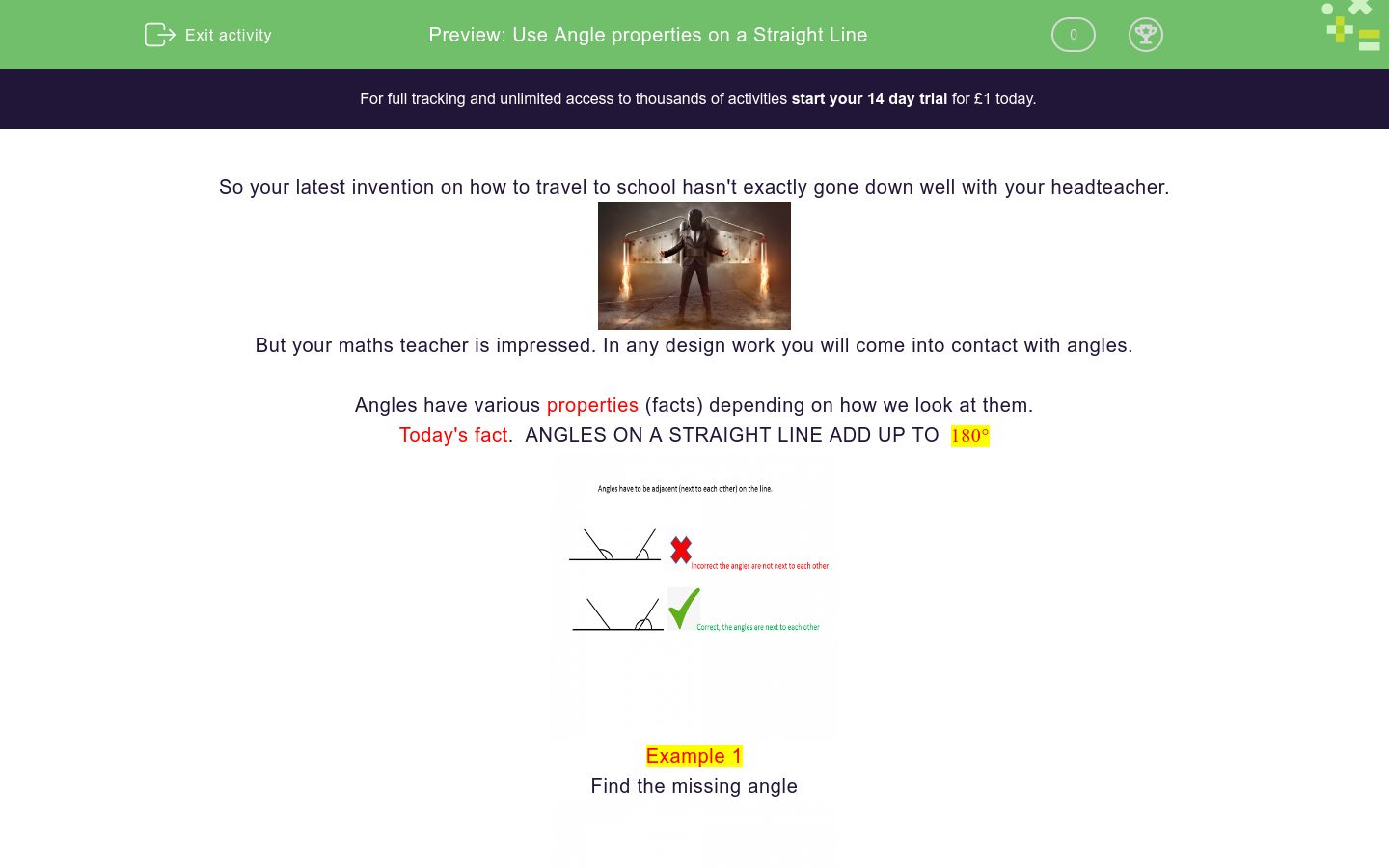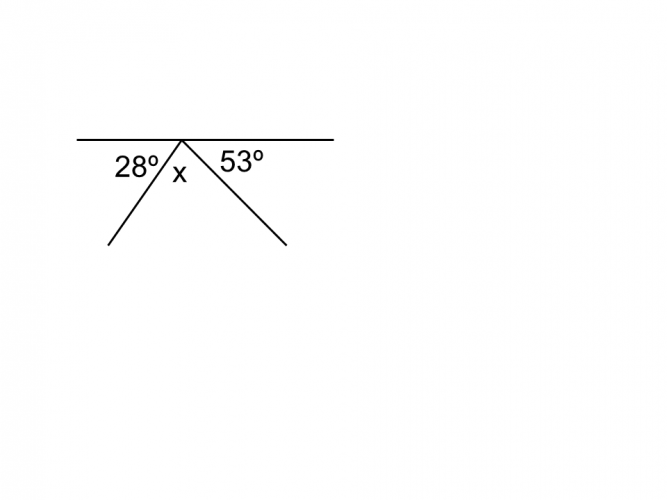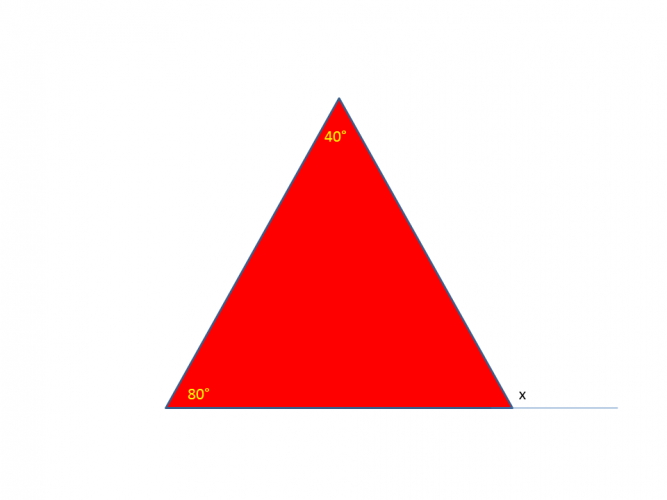# Use Angle properties on a Straight Line

In this worksheet, students will learn the rule about angles on a straight line and be able to calculate missing angles.Key stage:  KS 4

GCSE Subjects:   Maths

GCSE Boards:   AQA, Eduqas, OCR, Pearson Edexcel

Curriculum topic:   Geometry and Measures, Basic Geometry

Curriculum subtopic:   Properties and Constructions, Angles

Difficulty level:### QUESTION 1 of 10

So your latest invention on how to travel to school hasn't exactly gone down well with your headteacher.But your maths teacher is impressed. In any design work you will come into contact with angles.

Angles have various properties (facts) depending on how we look at them.

Today's fact.  ANGLES ON A STRAIGHT LINE ADD UP TO  180°Example 1

Find the missing angleWe know that the sum of the angles must add up to 180°

180° - 45° = 135°

Example 2We might have been given two angles but that is no bother.

28° - 53° = 81°

180° - 81° = 99°

Are you ready for lift off?Calculate the value of S.

140°

130°

150°

180°

Choose the correct options from the following

To find a missing angle on a straight line

Subtract the angle from 180°

Add the angles together and subtract from 180°What is the value of h?What is the value of the missing angle?What is the value of x?What is the value of x?

 120° 80° 40° 60° x is equal toThe three missing angles all have the same value.

What is that value?

The value of each missing angle is 108° 36° 72°Lets go for it.

What is the value of x?Let's try this.

What is the value of x?

• Question 1Calculate the value of S.

150°
EDDIE SAYS
We are lucky we only have to remember one number and that is 180. Subtract 30 from 180 to give us the missing angle. How easy is that..
• Question 2

Choose the correct options from the following

To find a missing angle on a straight line

Subtract the angle from 180°
Add the angles together and subtract from 180°
EDDIE SAYS
It is just a case of knowing the rule. Once you have got it..simple (like everything) Either subtract from 180 or if there is more than one angle add them together first and them subtract from 180.
• Question 3What is the value of h?

126
EDDIE SAYS
Just apply the rule, it really is that simple. Don't forget to show your working out. This is where you get the marks from as well as an answer. 180 - 54 = 126
• Question 4What is the value of the missing angle?

EDDIE SAYS
Just that little extra step. Add up your two angles first 86 + 22 = 108 180 - 108 = 72°
• Question 5What is the value of x?

48
EDDIE SAYS
And just when you thought it was safe. Angles on a straight line can appear in various shapes. Remember they have to be next to each other. The rest of the triangle can be ignored. 180 - 132 = 48°
• Question 6EDDIE SAYS
Are you getting the hang of this now. 180 - 111 = 69°
• Question 7What is the value of x?

 120° 80° 40° 60° x is equal to
EDDIE SAYS
Now I know this is a little sneaky. You can clearly see the angle on the straight line that you need to calculate. It is just that the angle next to it is missing and you need to find that first. You may recall that angles in a triangle also add up to 180° so find the missing angle first. 40 + 80 = 120° 180 - 120 = 60° now carry on as normal 180 - 60 = 120°
• Question 8The three missing angles all have the same value.

What is that value?

The value of each missing angle is 108° 36° 72°
EDDIE SAYS
Why work out one missing angle when you can find three!! 53 + 19 = 72° Now subtract from 180 180 - 72 = 108° You know the three missing angles are all the same. So share out 108 between three. 108 ÷ 3 = 36. Check 36 + 36 + 36 + 53 + 19 = 180°
• Question 9Lets go for it.

What is the value of x?

38
EDDIE SAYS
No need for panic on these. We have got this. First of all find out how many degrees we have to share with 4x. 180 - 28 = 152° Now share between 4. 152 ÷ 4 = 38°
• Question 10Let's try this.

What is the value of x?

33
EDDIE SAYS
180 - 15 = 165° We can see that in total there is 5x. Just take 165 ÷ 5 = 33° Don't let the lack of numbers put you off.
---- OR ----

Sign up for a £1 trial so you can track and measure your child's progress on this activity.

### What is EdPlace?

We're your National Curriculum aligned online education content provider helping each child succeed in English, maths and science from year 1 to GCSE. With an EdPlace account you’ll be able to track and measure progress, helping each child achieve their best. We build confidence and attainment by personalising each child’s learning at a level that suits them.

Get started